# Automatic coordination control technology of interconnected medium voltage direct current (MVDC) distribution network based on frequency deviation

## Kunyang Ji1

1Liaoyuan Vocational Technical College, Liaoyuan, Jilin, 136201, China

Journal of Vibroengineering, (in Press). https://doi.org/10.21595/jve.2022.22849
Received 4 August 2022; received in revised form 12 December 2022; accepted 26 December 2022; published 14 February 2023

Copyright © 2023 Kunyang Ji. This is an open access article distributed under the Creative Commons Attribution License, which permits unrestricted use, distribution, and reproduction in any medium, provided the original work is properly cited.
Views 5
CrossRef Citations 0
Abstract.

The automatic coordination control technology of interconnected medium voltage direct current (MVDC) distribution network based on frequency deviation is studied to improve the influence of frequency deviation on the operation of MVDC distribution network. Firstly, two regional interconnected MVDC distribution networks are built, and connected and operated in parallel with the AC power grid through the medium voltage single-phase AC port, to realize the interconnection of different regions of the distribution network according to the MVDC feeder. Through the mutual power support of each division of the distribution network, the frequency of each division of the distribution network is the same, and the frequency deviation of each division is reduced. According to the zoning frequency characteristics of the interconnected MVDC distribution network, the control strategy of the flexible interconnection device and the control strategy of the photovoltaic power generation system are combined to realize the automatic coordinated control of the frequency deviation of the interconnected MVDC distribution network. The experimental results show that this technology can improve the frequency deviation of the interconnected MVDC distribution network, realize the automatic coordinated control of the distribution network, and the fluctuation range of the tie line power deviation in each region decreases significantly.

Highlights
• In order to improve the influence of frequency deviation on the operation of interconnected medium voltage direct current (MVDC) distribution network, the automatic coordination control technology of interconnected medium voltage direct current distribution network based on frequency deviation is studied.
• An MVDC distribution network connected to two regions is built, and the AC power network is connected through medium-voltage single-phase AC ports to realize the interconnection and interworking of different regions of the distribution network according to the MVDC feeders.
• Through the mutual power support of each zone in the distribution network, the frequency of each zone is the same and the frequency deviation of each zone is reduced.

Keywords: frequency deviation, medium voltage direct current (MVDC), interconnected distribution network, automation, coordinated control technology, mutual power support.

#### 1. Introduction

Compared with the traditional AC distribution network, the DC distribution network constructed with flexible DC technology can save a lot of interconnected MVDC distribution network DC/AC converter links and improve the operation efficiency of the system; It can increase the power supply capacity and radius of the system and reduce the occupied area of urban land; It can reduce the electromagnetic radiation on the line [1-2] and reduce the interference to the communication system. In addition, it can also solve a series of power quality problems such as large line losses in the existing distribution network, voltage fluctuations, grid harmonics, three-phase imbalance and so on , and greatly improve the reliability and controllability of urban power distribution. Therefore, it is a power distribution method with great technical advantages and development potential. However, at present, the research on DC system mainly focuses on high-voltage DC transmission and low-voltage microgrid , and there is less research on medium voltage DC distribution network.

The feeders in the existing urban AC distribution network are connected through tie switches , which cannot realize the interconnection between regions and the flexible networking of distributed generation (DG). However, medium voltage direct current (MVDC) distribution technology has become an important direction for the development of distribution networks in the future with its advantages of long power supply radius, high operation efficiency and closed-loop operation . The power electronic transformer (PET) with medium / low voltage AC/DC multiple voltage ports can realize the flexible access and efficient matching of DC source and load, and provides the possibility for the realization of flexible distribution network based on MVDC interconnection. It is an effective carrier of energy routing in flexible interconnection distribution network in the future. Different from the traditional radial AC power supply mode, in the flexible interconnected MVDC distribution network, MVDC channels are built between adjacent regional subnets by power electronic conversion devices for interconnection and mutual supply , forming a multi terminal parallel supply and distribution network or ring distribution network. The power supply equipment also includes not only AC power supply, but also DC power supply such as DG, energy storage and electric vehicle charging station access. Compared with the AC distribution network system architecture, flexible interconnected MVDC distribution network can effectively reduce the design capacity and loss of traditional transformers, improve energy efficiency, the flexibility of DG, energy storage and electric vehicle charging station access and the controllability of the power grid , and solve the problem of equipment and line utilization decline caused by the dynamic imbalance of load distribution in time and space of the traditional power network. The access of energy storage system can enhance the rapid regulation ability of power grid to deal with uncertain events, and realize peak shaving and valley filling.

Electric energy is the core energy of national economic development. With the development of science and technology, the types and scale of electrical equipment are expanding rapidly, which also puts forward higher requirements for the stability and security of power supply. At present, the feeders of most power grid systems are connected through tie switches , and the balanced conversion of power supply power between different power supply areas cannot be realized, and flexible networking cannot be carried out, so the further development of scientific power supply is greatly limited.

At present, there are many researches on coordinated control of distribution network. Li et al. studied the load balance control of low-voltage distribution network to establish electrical , build a complete process framework of load balance control, design three-phase load balance control device, and achieve smooth commutation of single-phase load through functional units; The load distribution scheme is optimized by the load priority arrangement method of leaf nodes, so as to suppress the generation of harmonics and realize the stable distribution of current and voltage; The mathematical model of imbalance compensation is established to optimize the control effect of low-voltage distribution load balance and improve the load balance of distribution network; Mudalya et al. studied the coordinated control of low-voltage DC distribution network . This strategy estimates the current state of the network and generates a group of references for controllable sources, so as to minimize the load voltage deviation. This paper also proposes a soft open point (SOP) based on power electronic converter, which provides seamless connection of remote end and additional freedom to control circulating current between feeders. The detailed description and control strategy of SOP are given. The steady state analysis of the three feeder DDN is carried out with and without the proposed SOP. The operation reliability of low-voltage DC distribution network is improved. Wang et al. studied  a new hybrid coupled interconnection converter (HCIC) structure for hybrid AC and low-voltage DC (LVDC) microgrids, which is composed of a converter and a static var compensator (SVC) in series. In addition, a new droop control method is developed for the proposed HCIC. The basic active and reactive power flow in the hybrid AC/DC microgrid is determined by the P-$\delta$ and Q-P-V-$\alpha$ Droop control, and P-Q is proposed for SVC part of HCIC- $\alpha$ Droop controller. In addition, based on the decoupling control strategy, the fast harmonic control is given to improve the filtering characteristics of the SVC part of the HCIC, suppress harmonic power, and/or inject active power.

In view of the shortcomings of the existing power supply system and the demand for power supply flexibility of the power supply network in the expansion of urbanization, a new coordinated control technology of the interconnected MVDC distribution network is proposed. In this system, the medium voltage DC interconnection is taken as the core, and the interconnection channel is established between the power supply networks in different regions to realize the ring closed multi-terminal parallel power supply structure. This technology not only reduces the loss of current in the conversion process, but also realizes the complementary energy between different regions. The simulation analysis shows that the system can realize the balanced distribution of power according to the power situation between each port, which is of great significance to improve the operation reliability of the power supply system.

#### 2.1. Overall structure of interconnected MVDC distribution network

The overall structure of interconnected MVDC distribution network is shown in Fig. 1. As shown in Figure 1, the overall structure of the interconnected MVDC distribution network takes the interconnection of two regions as an example, adopts the two-terminal parallel power supply structure, and is formed through the transformation of the existing AC distribution network. The structure is connected to the AC power grid through the single-phase medium voltage AC (MVAC) port and operates in parallel. Inter-regional interconnection is realized based on MVDC feeders. The feeder adopts 20 kV (± 10 kV) voltage level, which is similar to the traditional 20kV AC distribution network in terms of power supply radius, and can meet the capacity requirements of the existing 10kV and 20kV AC distribution network, and can adapt to the further improvement of the DC distribution network capacity in the future. At the same time, the low-voltage DC (LVDC) port connects the DC load, energy storage system and photovoltaic in the area to form the LVDC microgrid . All devices in LVDC microgrid organize and work together by themselves, and the upper system does not interfere too much. Since the input voltage level of photovoltaic access is affected by maximum power point tracking (MPPT), most of them choose 750 V to 1 kV, so the LVDC port voltage level is set at 760 V (± 380 V).

Fig. 1. Overall structure of the MVDC interconnected distribution network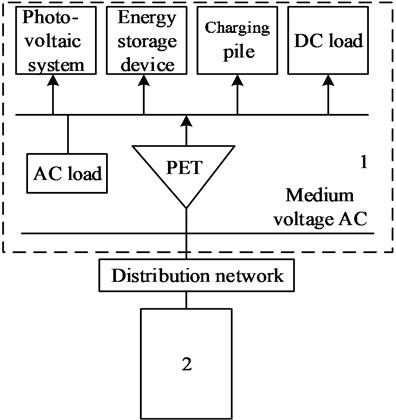When the regional power supply system is in a steady state, the power grid connected to each port is actively controlled, and the energy of the two regional distribution networks is evenly distributed through MVDC feeders to balance the energy difference in the region. At the same time, the dispersed micro energy nodes in the region can form a “chain” or “ring” through the LVDC microgrid to exchange energy and optimize power flow within or between regions. When the sudden increase of load in a certain area or the shortage of AC power supply lead to a gap in the power supply in this area, the energy of adjacent areas can support the gap area through MVDC feeder  to supply power for its load, so that the power supply systems between the two areas can achieve the role of “mutual standby” and “mutual peak valley support”. This method not only provides uninterrupted and stable power supply for the load, but also effectively reduces the startup and shutdown times of the generator set, reduces the investment cost of the installed capacity of the system and various losses in the power grid. Finally, an integrated flexible interconnected distribution network with multi terminal connection of lines, flexible load transfer and strong grid structure can be formed.

#### 2.2. Influence of grid frequency deviation on active frequency of interconnected MVDC distribution network

According to the power angle characteristics of synchronous generator in interconnected MVDC distribution network, when the output impedance of virtual synchronous generator is inductive, the output active power is directly proportional to the power angle of synchronous generator. ${w}_{g}$ represents the angular frequency of the interconnected MVDC distribution network, ${K}_{p}\approx 2{U}_{c}{U}_{g}/X$ represents the active power regulation coefficient, $X$ represents the synchronous impedance of the interconnected MVDC distribution network, and ${U}_{c}$ and ${U}_{g}$ represent the inverter output phase voltage and grid phase voltage amplitude of the interconnected MVDC distribution network respectively. The closed-loop transfer function expression of output active power and active power command value and grid frequency deviation value in interconnected MVDC distribution network can be obtained as follows:

(1)
${P}_{out}={G}_{P-{P}_{ref}}{P}_{ref}+{G}_{p-\mathrm{\Delta }w}{P}_{ref}\left({w}_{0}-{w}_{g}\right),$

where, ${w}_{0}$ represents the rated angular frequency, ${G}_{P-{P}_{ref}}$ and ${G}_{p-\mathrm{\Delta }w}$ represent the adjustment coefficients of the frequency deviation of the active power respectively , and their expression is as follows:

(2)
${G}_{P-{P}_{ref}}=\frac{{w}_{0}{K}_{p}}{{J}_{w}{w}_{0}{s}^{2}+{K}_{w}+{D}_{w}s+{K}_{p}},$
(3)
${G}_{p-\Delta w}=\frac{{K}_{p}\left({J}_{w}{w}_{0}s+{D}_{w}\right)+{K}_{w}}{{J}_{w}{w}_{0}{s}^{2}+{K}_{w}+{D}_{w}s+{K}_{p}},$

where, ${D}_{w}$ and ${J}_{w}$ represent the damping coefficient and moment of inertia of the virtual synchronous motor respectively.

The closed-loop transfer function expression of the output frequency of the traditional virtual synchronous generator, the active power command value and the grid frequency deviation value in the interconnected MVDC distribution network can be obtained:

(4)
$w={G}_{w-{P}_{ref}}{P}_{ref}-{G}_{w-\mathrm{\Delta }w}\left({w}_{0}-{w}_{g}\right),$

where, ${G}_{w-{P}_{ref}}$ and ${G}_{w-\mathrm{\Delta }w}$ respectively represent the adjustment coefficient of the frequency deviation of reactive power , and its expression is as follows:

(5)
${G}_{w-{P}_{ref}}=\frac{{w}_{0}s}{{J}_{w}{w}_{0}{s}^{2}+{K}_{w}+{D}_{w}s+{K}_{p}},$
(6)
${G}_{w-\Delta w}=\frac{{w}_{0}{K}_{p}}{{J}_{w}{w}_{0}{s}^{2}+{K}_{w}+{D}_{w}s+{K}_{p}}.$

Assuming that the power quality in the interconnected MVDC distribution network is good, $w={w}_{g}-{w}_{0}$ is considered in this case, so in the steady state, the output active power of the interconnected MVDC distribution network is equal to the command value.

In the actual operation of the interconnected MVDC distribution network, the grid frequency of the interconnected MVDC distribution network cannot be guaranteed to be the standard frequency. When $\mathrm{\Delta }w=0$, the output active power is only related to ${G}_{p-{P}_{ref}}$, and the output frequency is only related to ${G}_{w-{P}_{ref}}$. According to the existing conclusions, ${J}_{w}$, ${D}_{w}$ and other parameters only affect the dynamic characteristics of ${G}_{p-{P}_{ref}}$ and ${G}_{w-{P}_{ref}}$, but do not affect the steady-state value. Therefore, when $\mathrm{\Delta }w=0$, the output active power and frequency can be tracked without error. When $\mathrm{\Delta }w\ne 0$, the output frequency of the interconnected MVDC distribution network is jointly determined by ${G}_{w-{P}_{ref}}$ and ${G}_{w-∆w}$, and the role of ${G}_{w-∆w}$ cannot be ignored. Comparing the transfer function, it can be found that ${G}_{w-∆w}={G}_{p-{P}_{ref}}$, both of which have the same dynamic and steady-state characteristics. Therefore, the existence of ${G}_{w-∆w}$ changes the dynamic process of the output frequency of the interconnected MVDC distribution network. If the parameters when $\mathrm{\Delta }w=0$ are still used, it may lead to large overshoot in the dynamic process, and makes the dynamic output frequency of the virtual synchronous generator out of limit. The output active power is jointly determined by ${G}_{p-{P}_{ref}}$ and ${G}_{p-∆w}$, and the characteristics of ${G}_{p-{P}_{ref}}$ are consistent with that of $\mathrm{\Delta }w=0$. The overshoot and adjustment time of the dynamic process of ${G}_{p-∆w}$ increase with the increase of ${J}_{w}$, and its adjustment time is also affected by ${D}_{w}$ and decreases with the increase of ${D}_{w}$, but its overshoot is less affected by ${D}_{w}$. If the parameter setting is unreasonable, it may lead to the overshoot of the output active power and the shutdown of the inverter. At the same time, ${D}_{w}$ affects the steady-state characteristics of ${G}_{p-∆w}$, so the existence of ${G}_{p-∆w}$ makes the output active power unable to realize the zero error tracking of the command value. The steady-state value of active power is :

(7)
${P}_{out}={P}_{ref}+{K}_{w}∆w+{D}_{w},$

where, the value of ${K}_{w}$ is large, so that the deviation between ${P}_{out}$ and ${P}_{ref}$ cannot be ignored, and the smaller the capacity of the virtual synchronous generator or the larger the value of ${D}_{w}$ is, the more serious the impact of the deviation between the two is. When $\mathrm{\Delta }w<0$, ${P}_{out}<{P}_{ref}$, the operation efficiency of the virtual synchronous generator will be reduced. When $\mathrm{\Delta }w>0$, ${P}_{out}>{P}_{ref}$, the virtual synchronous generator needs to send more power, which is easy to cause the overload of the virtual synchronous generator. The active power deviation rate is defined here to reflect the deviation degree between the output frequency of the output power and the given value. The expression of the active power deviation rate is as follows :

(8)
$\lambda ={K}_{w}∆w+{D}_{w}/{P}_{ref}.$

When the frequency of the interconnected MVDC distribution network deviates, by changing the operating parameters of the interconnected MVDC distribution network, the overshoot and dynamic response time of the output active power and frequency of the interconnected MVDC distribution network can meet the requirements, while the steady-state value of the active power does not change with the parameters, so as to realize the zero error control of the active power of the interconnected MVDC distribution network.

#### 2.3. Zoning frequency characteristics of interconnected MVDC distribution network

The frequency deviation adjustment of interconnected MVDC distribution network is realized by using the zonal frequency characteristic analysis of interconnected MVDC distribution network. The sectional interconnection structure diagram of interconnected MVDC distribution network is shown in Fig. 2. It can be seen from Fig. 2 that the AC power grid before the partition of the interconnected MVDC distribution network is a receiving power grid with multiple DC feeds, which faces problems such as excessive short-circuit current and simultaneous commutation failure of multiple DC circuits . Using the back-to-back technology of the interconnected MVDC distribution network, the receiving end power grid is divided into multiple asynchronous interconnected partitions. The coordinated control of active power between each partition is the key to ensure the safe and stable operation of the interconnected MVDC distribution network.

Fig. 2. Partition interconnection structure diagram of interconnected MVDC distribution network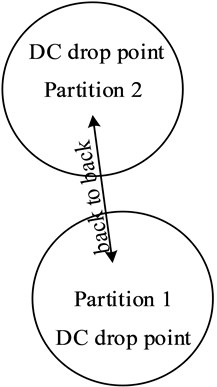When the interconnected MVDC distribution network is in the steady-state operation state, each partition is interconnected asynchronously, and the active power transmitted between each partition receives the dispatching instruction, so as to ensure that the frequency between each partition is maintained at the rated frequency. Once a power disturbance occurs, for example, a power disturbance $\mathrm{\Delta }P$ occurs in area 1, assuming that the transmitted power between zones does not change, for area 1 of the interconnected MVDC distribution network, there is an expression as follows:

(9)
$\mathrm{\Delta }P=-{K}_{S1}\mathrm{\Delta }{f}_{1},$

where, ${K}_{S1}$ is the regulated power of unit frequency of area 1 (integrating the frequency characteristics of generator and load); $\mathrm{\Delta }{f}_{1}$ is the deviation between the frequency of area 1 and the rated frequency; $\mathrm{\Delta }P$ is the power disturbance in area 1 of the interconnected MVDC distribution network.

According to Eq. (9), if there is no mutual power support between partitions of the interconnected MVDC distribution network, a large frequency deviation will occur in the frequency of this partition after the power disturbance in one partition , affecting the frequency stability of this partition. Therefore, it is necessary to quickly adjust the back-to-back power of the interconnected MVDC distribution network to make the frequencies of each partition equal, that is, after the power disturbance, the interconnection partitions of all interconnected MVDC distribution networks cooperate with each other to jointly bear the power disturbance. The existing expression is as follows:

(10)
$\mathrm{\Delta }P=-{K}_{S1}\mathrm{\Delta }{f}_{1}-{K}_{S2}\mathrm{\Delta }{f}_{2},$

where ${K}_{Si}$ is the regulated power of unit frequency of zone $i$ (integrating the frequency characteristics of generator and load); $\mathrm{\Delta }{f}_{i}$ is the deviation between the frequency of zone $i$ of interconnected MVDC distribution network and the rated frequency.

The most ideal frequency response of the partition interconnection of the interconnected MVDC distribution network is that the frequency of each partition is the same, that is, all the asynchronous interconnected partitions of the interconnected MVDC distribution network respond to the power disturbance together like the “synchronous network”, so that the frequency of each partition of the interconnected MVDC distribution network is the same, and the expression is as follows:

(11)
$\mathrm{\Delta }{f}_{1}=\mathrm{\Delta }{f}_{2}=\mathrm{\Delta }f.$

According to simultaneous Eqs. (10) and (11), it can be obtained:

(12)
$\mathrm{\Delta }f=-\frac{\mathrm{\Delta }P}{{K}_{S1}+{K}_{S2}}.$

The power disturbance share assumed by zone $i$ is expressed as follows:

(13)
$\mathrm{\Delta }{P}_{i}=-{K}_{Si}\mathrm{\Delta }f={K}_{Si}\frac{\mathrm{\Delta }P}{{K}_{S1}+{K}_{S2}}.$

During the operation of the interconnected MVDC distribution network, once the power disturbance occurs in area 1, the expression of the mutual active power urgently transmitted from area 2 of the interconnected MVDC distribution network to area 1 is as follows:

(14)
$\mathrm{\Delta }{P}_{ij}=-{K}_{Si}\mathrm{\Delta }f=\frac{{K}_{Si}}{{K}_{S1}+{K}_{S2}},$

where, $\mathrm{\Delta }{P}_{ij}$ is the active power increment transmitted from zone $i$ to zone $j$. Once the power disturbance $\mathrm{\Delta }P$ occurs in area 1 of the interconnected MVDC distribution network, and the adjacent area 2 provides emergency power support for area 1 as Eq. (14), the power disturbance borne by area 1 of the interconnected MVDC distribution network is as follows:

(15)
$\mathrm{\Delta }{P}_{i}=\mathrm{\Delta }P-\mathrm{\Delta }{P}_{21}=\frac{{K}_{S1}}{{K}_{S1}+{K}_{S2}}\mathrm{\Delta }P.$

Then the frequencies of area 1 and area 2 are the frequencies of Eq. (12), so as to minimize the frequency deviation of the zone interconnection system of the entire interconnected MVDC distribution network.

#### 2.4. Automatic coordination control of interconnected MVDC distribution network with frequency deviation

According to the zoning frequency characteristics of the interconnected MVDC distribution network, the control strategy of the flexible interconnection device and the control strategy of the photovoltaic power generation system are combined to realize the automatic coordination control of the frequency deviation of the interconnected MVDC distribution network.

#### 2.4.1. Control strategy of flexible interconnection device

The flexible interconnection device of the interconnected MVDC distribution network mainly includes layered DC droop control, constant voltage and constant frequency control and current limiting control. The control structure diagram of the flexible interconnection device of the interconnected MVDC distribution network is shown in Fig. 3.

Fig. 3. Control structure diagram of flexible interconnection device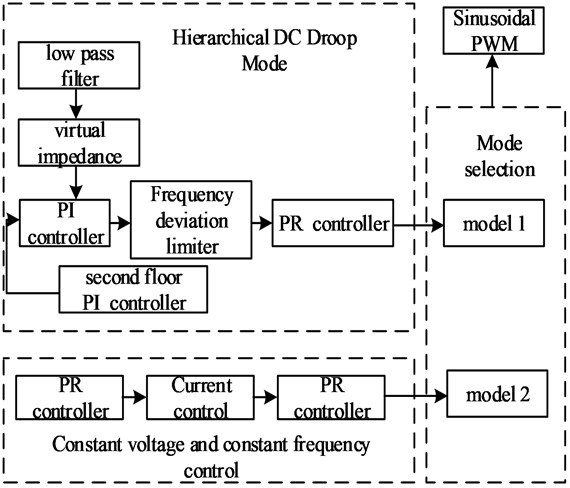During the normal operation of the interconnected MVDC distribution network, the flexible interconnection device of the interconnected MVDC distribution network adopts layered DC droop control, and the DC droop control equation is:

(16)
${U}_{dc}^{\mathrm{*}}={U}_{dcN}-{k}_{p}{i}_{dc}-\mathrm{\Delta }{P}_{i},$

where ${U}_{dc}^{\mathrm{*}}$ is the reference value of DC voltage ${U}_{dc}$; ${k}_{p}$ is the virtual impedance; ${i}_{dc}$ is DC current; ${U}_{dcN}$ is the rated DC voltage.

The inconsistent distance between the interconnection devices of the interconnected MVDC distribution network makes its line impedance not exactly the same, which leads to the difference in the output voltage at each end of the interconnected MVDC distribution network. The addition of virtual impedance is usually used to suppress the influence of the inconsistent line impedance. Large virtual impedance of interconnected MVDC distribution network will cause DC voltage drop. In order to compensate for the voltage drop, a two-layer control strategy based on low bandwidth communication technology is adopted, in which the compensation voltage is:

(17)
$\mathrm{\Delta }{u}_{dc}=\left({U}_{dcN}-{U}_{dc}\right)\left(\frac{{k}_{p}+{k}_{i}}{s}\right)-\mathrm{\Delta }{P}_{i},$

where, $\mathrm{\Delta }{u}_{dc}$ is the output value of the two-layer controller of the interconnection device in the interconnected MVDC distribution network; ${k}_{p}$ is the proportion coefficient; ${k}_{i}$ is the integral coefficient.

The compensation voltage is superimposed on the droop control to generate the reference value, and the voltage reference value is subtracted from the feedback value to obtain the current inner loop reference value ${I}_{\alpha \beta }^{\mathrm{*}}$ through the proportion-integral regulator. The current closed loop adopts the control strategy under the $\alpha \beta$ coordinate system, and the proportion-resonant PR regulator is used to realize the static error free regulation.

When a three-phase short-circuit fault occurs on the AC side of the interconnection device of the interconnected MVDC distribution network, the interconnection device is switched from layered droop control to constant voltage and constant frequency control . The constant voltage and constant frequency control in $\alpha \beta$ coordinate system is adopted, the AC voltage reference value ${U}_{\alpha \beta }^{\mathrm{*}}$ is set as two-phase sinusoidal quantity, and the proportion-resonance regulator is also used to realize no static error regulation.

When the interconnected converters of the interconnected MVDC distribution network adopt different control strategies, frequency deviation limiting links (the upper and lower limits are ${f}_{\mathrm{m}\mathrm{a}\mathrm{x}}$ and ${f}_{\mathrm{m}\mathrm{i}\mathrm{n}}$ respectively) are added to the control block diagram to ensure the stable and reliable switching of the interconnected MVDC distribution network, so as to avoid the over-current phenomenon that triggers the protection link and leads to the shutdown of the equipment of the interconnected MVDC distribution network in the switching process of different control strategies of the interconnected MVDC distribution network.

#### 2.4.2. Control strategy of photovoltaic power generation system

The control strategy of photovoltaic power generation system in interconnected MVDC distribution network includes maximum power point tracking control and layered DC droop control. Its control block diagram is shown in Fig. 4.

Fig. 4. Control structure diagram of photovoltaic power generation system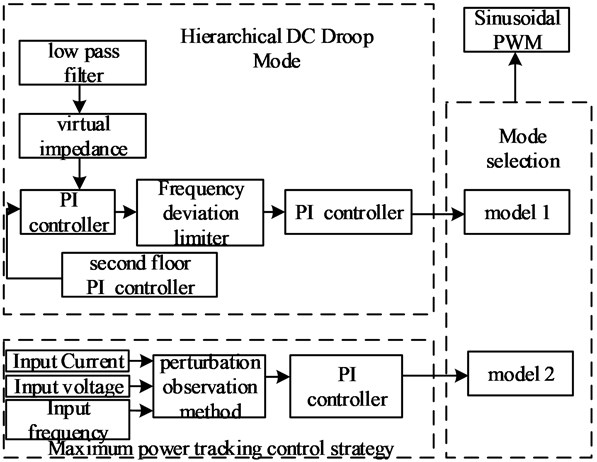During normal operation, the photovoltaic power generation unit works in the maximum power point tracking mode, and the layered droop control is mainly started when the SOC value of the battery reaches the upper limit. At this time, because the energy storage device stops working, in order to maintain the constant state of DC bus voltage and the output frequency of the distribution network, multiple photovoltaic power generation units are switched to the layered DC droop control at the same time to reduce the output power of the photovoltaic power generation unit. In order to prevent the output current from exceeding the limit, a limiting module is added to the control system to ensure the stable switching of the interconnected MVDC distribution network under different control modes.

#### 3. Results

In order to verify the correctness and effectiveness of the proposed scheme, the structure of interconnected MVDC distribution network with full voltage level is established by using MATLAB simulation analysis software, and a simulation model of 10 kW interconnected MVDC distribution network is built. The main parameters of the interconnected MVDC distribution network are shown in Table 1.

Table 1. Simulation parameters of MV DC interconnected distribution network

 Indicator name Unit Numerical value Rated line voltage rad/s 380 Rated angular frequency mH 110$\pi$ Rated inductance μF 5 Rated capacitance kHz 25 On-off level kV 6 DC side voltage V 0.85 Damping coefficient N/(m/s) 11$\pi$ Active power regulation factor – 5200

The simulation analysis of the regulation reliability during the operation of the constructed interconnected MVDC distribution network is carried out. The results are shown in Fig. 5 and Fig. 6. Positive values in the figure indicate that the power inflows are from the port of the interconnected MVDC distribution network, and negative values indicate that the power flows from the port of the interconnected MVDC distribution network.

Fig. 5. Area 1 port power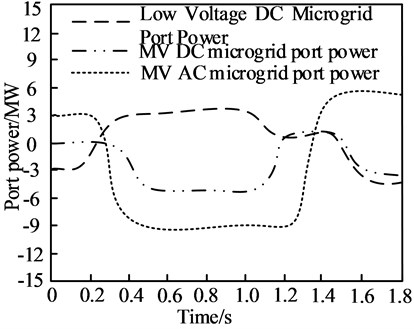Fig. 6. Area 2 port power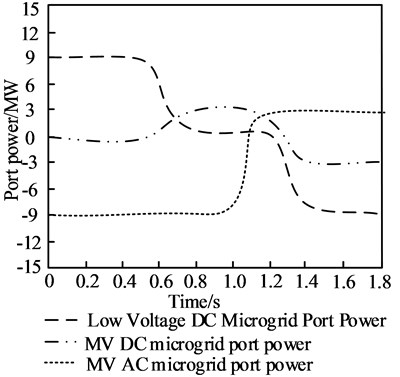From the actual simulation analysis results, it can be seen that within 0-0.4 s, the system in area 1 can also absorb the surplus power in the microgrid through the low-voltage DC port, and then supply the power load, while ensuring the power required for the operation of the low-voltage DC power grid. Within the range of 0.4-0.8 s, the power load in area 1 begins to rise, and the system begins to have a 5 MW power gap. At this time, the power supply network in area 2 begins to provide balanced power to area 1 through the medium voltage DC interconnected distribution system. Within the range of 0.8-1.2 s, there is still a power gap of about 2.5 MW for the electrical load in region 1. At the same time, the distribution network in area 2 continues to output the same amount of power to area 1, thus effectively filling the power gap during the operation of the microgrid. Within the range of 1.2-1.6 s, there is a 2.5 MW power gap in the power load in area 2. At this time, area 1 starts to output power to area 2 through the network to ensure the balance of power in the system. The simulation analysis shows that the interconnected MVDC distribution coordination control technology can realize the balanced distribution of power according to the power situation between each port, reduce the energy consumption in the process of power supply, and improve the reliability and economy of power supply.

The technology in this paper is used to automatically coordinate and control the interconnected MVDC distribution network. The load frequency control effect in different areas of the interconnected distribution network is counted, and the frequency deviation of output in different areas is counted. The statistical results are shown in Fig. 7.

Fig. 7. Frequency deviation control results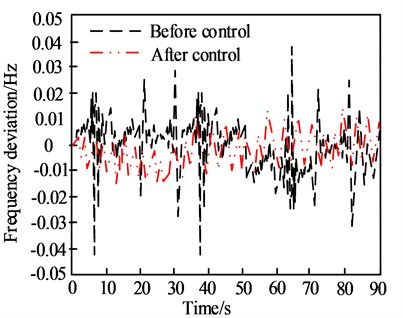a) Area 1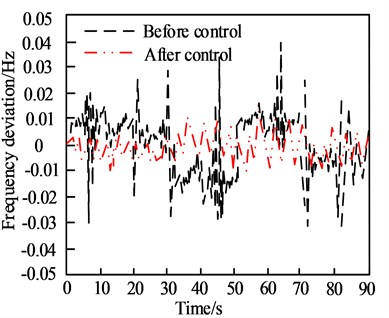b) Area 2

From the experimental results in Fig. 7, it can be seen that using this technology to automatically coordinate and control the interconnected MVDC distribution network can effectively reduce the frequency deviation in different areas of the interconnected MVDC distribution network and stabilize the frequency deviation of the interconnected MVDC distribution network within the ideal range.

The technology in this paper is used to automatically coordinate and control the interconnected MVDC distribution network. Before and after control by using the method in this paper, the power change results at the tie line of the interconnected MVDC distribution network are shown in Fig. 8.

Fig. 8. Power deviation at the tie line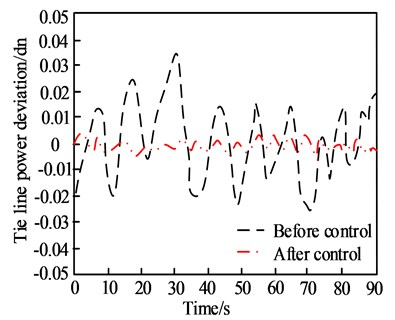According to the experimental results in Fig. 7 and Fig. 8, under the random wind interference of the interconnected MVDC distribution network, the technology in this paper can effectively control the load frequency of the two regional interconnected power grids in the interconnected MVDC distribution network. The frequency deviation of the load frequency of the two regions in the interconnected MVDC distribution network after automatic coordination control is significantly lower than that before automatic coordination control. The fluctuation range of frequency deviation in the first two areas of automatic coordinated control is large. After using this technology to automatically coordinate and control the interconnected MVDC distribution network, the fluctuation range of frequency deviation in the two areas is significantly reduced; Before using this technology to automatically coordinate and control the interconnected MVDC distribution network, the fluctuation range of the power deviation of the tie lines in the two regions is large. After using this technology to automatically coordinate and control the interconnected MVDC distribution network, the fluctuation range of the power deviation of the tie lines in the two regions decreases significantly. The experimental results show that this technology can automatically coordinate and control the frequency deviation of interconnected MVDC distribution network, and reduce the frequency deviation and tie line power deviation of interconnected MVDC distribution network.

#### 4. Discussion

This paper studies the automatic coordination control technology of interconnected MVDC distribution network based on frequency deviation, and realizes the automatic coordination control of MVDC distribution network. After entering the 21st century, with the large-scale construction of cities, the rapid development of enterprises, and the transformation of rural urbanization, the power load has increased rapidly, and the energy crisis and environmental crisis have become increasingly prominent. With the popularization of distributed energy and the development of high-end and sophisticated industries, people's requirements for the transmission capacity, power quality and power supply reliability of the distribution network are also increasing, making the existing power supply/distribution system gradually unable to meet the growing demand for power supply. The development of modern distribution network mainly has the following problems:

1) The distribution network corridor is tight, and the power supply capacity (power supply radius) is insufficient, so the power supply capacity needs to be expanded.

Under the same voltage level, the same floor area and cost, the transmission capacity of single circuit extruded polymer DC cable line is 1.5-2 times that of AC line, which can effectively improve the power supply capacity, expand the power supply radius and save land.

2) With the development of high-end technology industry, the demand for power quality and power supply reliability continues to increase.

The occurrence of power quality transient events (such as voltage fluctuations, flicker shocks, frequency changes, harmonics, etc.) has a serious impact on the manufacturing of chips, the welding of auto parts, the operation of CNC machine tools and other enterprises with high power quality needs. However, the DC distribution network can isolate the voltage drop of the AC system and control harmonics through the converter. According to statistics, under the same impact load, the voltage fluctuation can be reduced from ± 5 % guaranteed by the AC distribution network to ± 1 %-2 % by using the DC distribution network. At the same time, compared with the traditional AC distribution network, the flexible DC distribution network has less technical difficulty in realizing distributed energy storage. Once the battery energy storage technology breaks through the technical bottleneck, it can be widely configured in the DC distribution network, so as to better improve the power quality and the fault ride through capability of the system.

3) The proportion of distributed power generation, energy storage and DC load is increasing.

With the development of power electronic technology, more and more distributed power sources and energy storage devices will be included in the modern distribution network. For photovoltaic, although it generates DC power, it is unstable and cannot be used directly, and it still needs to undergo DC-DC and DC-AC conversion to access the AC distribution network; Although the wind power generates AC, it is not stable, and it needs AC-DC and DC-AC conversion to connect to the AC distribution network. If it is connected to the DC distribution network, the above DC-AC link can be omitted, and the phase and frequency of the voltage do not need to be locked, so the controllability and reliability can be further improved. As for the load, at present, the proportion of DC loads such as computers, mobile phones, tablet computers, electric vehicles, LED lighting, refrigerators, washing machines, variable frequency air conditioners and data centers is constantly increasing. In the AC distribution system, the AC-DC-AC conversion must be used to supply power to variable frequency equipment, and the AC-DC conversion must be used to supply power to DC loads. If the DC distribution network is adopted, the frequency conversion equipment only needs DC-AC conversion, and the DC load can be used directly, thus omitting a large number of AC-DC links, which not only reduces the access cost of each power supply and load, but also improves the efficiency of power use. Foreign studies estimate that 50 % of the load will need DC power supply in the next 20 years.

4) The line loss of AC distribution network is large, and the AC substation has electromagnetic radiation.

When the DC line voltage of the system is twice that of the AC system, the AC system will produce active loss due to the eddy current in the metal sheath of the cable. With the reactive loss of its own AC system, the line loss of the DC distribution network is only 15 %-50 % of that of the AC network. Although the AC system can reduce the line loss through reactive power compensation, it will increase the construction cost and complexity of the system. For the AC system with long lines, it is still unable to eliminate the restriction of power angle on transmission capacity in the power stability limit formula. AC substations in cities are prone to produce electromagnetic radiation to surrounding residents and interfere with radio communication. The “space charge effect” of DC lines makes corona loss and electromagnetic radiation smaller and has more environmental advantages.

5) The utilization of distribution equipment in AC distribution network is low.

The flexible DC technology is used to transform the traditional AC distribution network, so that the main transformers in the hybrid power grid are standby for each other, which greatly reduces the standby capacity of a single main transformer, saves costs, and improves system efficiency.

In addition, it can also provide a more “friendly” interface for the access of wind power, photovoltaic, energy storage and electric vehicles. Due to the flexible DC technology, the controllability and reliability of the system are greatly improved. From the above analysis, it can be seen that the traditional AC distribution network faces many problems and challenges, while the DC distribution network has the advantages of large transmission capacity, small transmission loss rate, low cable cost, high power supply quality, flexible and friendly access, flexible control and so on. Therefore, it is of great significance to study the DC distribution network.

#### 5. Conclusions

For the existing power supply system cannot form power balance and poor regulation performance in multi-port power supply, an automatic coordination control technology of interconnected MVDC distribution network based on frequency deviation is proposed. The automatic coordination control technology studied is applied to the interconnected MVDC distribution network. Through the experiment of the automatic coordination control technology, it is verified that the technology has the function of automatic coordination control of interconnected MVDC distribution network, with simple structure and strong adaptability. This technology can improve the poor regulation performance of the interconnected MVDC distribution network. By adjusting the frequency deviation of the interconnected MVDC distribution network, the good coordination of the interconnected MVDC distribution network can be realized, and the stable operation of the medium voltage DC distribution network can be realized.

#### Acknowledgements

The author received no funding for this work.

1. H. He, E. Du, N. Zhang, C. Kang, and X. Wang, “Enhancing the power grid flexibility with battery energy storage transportation and transmission switching,” (in Chinese), Applied Energy, Vol. 290, No. 2, p. 116692, May 2021, https://doi.org/10.1016/j.apenergy.2021.116692 [Publisher]
2. Y. Zhang, C. Liao, Y. Shang, W. Du, R. Huan, and Z. Ye, “Analysis of the field-line coupling of the transmission line above an orthogonal ground plane through electromagnetic reciprocity theorem,” (in Chinese), IEEE Transactions on Electromagnetic Compatibility, Vol. 62, No. 2, pp. 580–588, Apr. 2020, https://doi.org/10.1109/temc.2019.2944832 [Publisher]
3. B. Singh and P. Shukl, “Control of grid fed pv generation using infinite impulse response peak filter in distribution network,” IEEE Transactions on Industry Applications, Vol. 56, No. 3, pp. 3079–3089, May 2020, https://doi.org/10.1109/tia.2020.2968287 [Publisher]
4. L. Yang, J. Yang, M. Gao, Y. Chen, and X. Zhang, “A Systematic Approach via IIR Filters for Enhancing the Robustness of LCL-Type Shunt Active Power Filters to Grid Impedance,” (in Chinese), IEEE Transactions on Industry Applications, Vol. 56, No. 5, pp. 5095–5107, Sep. 2020, https://doi.org/10.1109/tia.2020.2999273 [Publisher]
5. D. Amarnath and S. Sujatha, “Internet-of-things-aided energy management in smart grid environment,” The Journal of Supercomputing, Vol. 76, No. 4, pp. 2302–2314, Apr. 2020, https://doi.org/10.1007/s11227-018-2492-5 [Publisher]
6. Y. Ji et al., “Overall control scheme for VSC‐based medium‐voltage DC power distribution networks,” (in Chinese), IET Generation, Transmission and Distribution, Vol. 12, No. 6, pp. 1438–1445, Mar. 2018, https://doi.org/10.1049/iet-gtd.2017.0912 [Publisher]
7. B. Zhao, Q. Song, J. Li, W. Liu, G. Liu, and Y. Zhao, “High-frequency-link dc transformer based on switched capacitor for medium-voltage dc power distribution application,” (in Chinese), IEEE Transactions on Power Electronics, Vol. 31, No. 7, pp. 1–1, 2015, https://doi.org/10.1109/tpel.2015.2483543 [Publisher]
8. Y. Wang, Q. Song, Q. Sun, B. Zhao, J. Li, and W. Liu, “Multilevel MVDC link strategy of high-frequency-link dc transformer based on switched capacitor for MVDC power distribution,” (in Chinese), IEEE Transactions on Industrial Electronics, Vol. 64, No. 4, pp. 2829–2835, Apr. 2017, https://doi.org/10.1109/tie.2016.2643622 [Publisher]
9. B. Bao, M. Lallart, and D. Guyomar, “Manipulating elastic waves through piezoelectric metamaterial with nonlinear electrical switched dual-connected topologies,” International Journal of Mechanical Sciences, Vol. 172, No. 36, p. 105423, Apr. 2020, https://doi.org/10.1016/j.ijmecsci.2020.105423 [Publisher]
10. J. Li and B. Du, “Intelligent Building Electrical Low Voltage Distribution Load Balance Control Method,” (in Chinese), Computer Simulation, Vol. 38, No. 4, pp. 73–77, 2021. [Search CrossRef]
11. S. Mudaliyar and S. Mishra, “Real-time coordinated control of low-voltage dc distribution network with soft opening point,” IEEE Transactions on Power Electronics, Vol. 36, No. 6, pp. 7123–7137, Jun. 2021, https://doi.org/10.1109/tpel.2020.3037020 [Publisher]
12. L. Wang, X. Fu, and M.-C. Wong, “Operation and control of a hybrid coupled interlinking converter for hybrid AC/Low voltage DC microgrids,” (in Chinese), IEEE Transactions on Industrial Electronics, Vol. 68, No. 8, pp. 7104–7114, Aug. 2021, https://doi.org/10.1109/tie.2020.3001802 [Publisher]
13. Y. Zeng, G. Zou, X. Zhang, X. Wei, L. Jiang, and C. Sun, “Faulty feeder selection and segment location method for SPTG fault in radial MMC‐MVDC distribution grid,” (in Chinese), IET Generation, Transmission and Distribution, Vol. 14, No. 2, pp. 223–233, Jan. 2020, https://doi.org/10.1049/iet-gtd.2018.6934 [Publisher]
14. S. Liu et al., “Practical method for mitigating three-phase unbalance based on data-driven user phase identification,” (in Chinese), IEEE Transactions on Power Systems, Vol. 35, No. 2, pp. 1653–1656, Mar. 2020, https://doi.org/10.1109/tpwrs.2020.2965770 [Publisher]
15. M. Abbasi and J. Lam, “A bridgeless ac/dc high voltage gain converter with three-phase modular series-output connected configuration for MVDC grid applications,” IEEE Transactions on Power Electronics, Vol. 35, No. 10, pp. 10323–10337, Oct. 2020, https://doi.org/10.1109/tpel.2020.2977912 [Publisher]
16. L. Chen et al., “Investigation of a modified flux-coupling-type SFCL for low-voltage ride-through fulfillment of a virtual synchronous generator,” (in Chinese), IEEE Transactions on Applied Superconductivity, Vol. 30, No. 4, pp. 1–6, Jun. 2020, https://doi.org/10.1109/tasc.2020.2970628 [Publisher]
17. M. Wu, J. Li, Q. Liu, S. Yang, and M. Molinas, “Measurement of impedance-frequency property of traction network using cascaded h-bridge converters: device design and on-site test,” (in Chinese), IEEE Transactions on Energy Conversion, Vol. 35, No. 2, pp. 746–756, Jun. 2020, https://doi.org/10.1109/tec.2020.2967055 [Publisher]
18. M. Jiang, Q. Guo, H. Sun, and H. Ge, “Short-term voltage stability-constrained unit commitment for receiving-end grid with multi-infeed hvdcs,” (in Chinese), IEEE Transactions on Power Systems, Vol. 36, No. 3, pp. 2603–2613, May 2021, https://doi.org/10.1109/tpwrs.2020.3031021 [Publisher]
19. K. Jia, Q. Zhao, T. Feng, and T. Bi, “Distance protection scheme for dc distribution systems based on the high-frequency characteristics of faults,” (in Chinese), IEEE Transactions on Power Delivery, Vol. 35, No. 1, pp. 234–243, Feb. 2020, https://doi.org/10.1109/tpwrd.2019.2909130 [Publisher]
20. M. Moradian, J. Soltani, G. R. Arab Markadeh, H. Shahinzadeh, and Y. Amirat, “A new grid-connected constant frequency three-phase induction generator system under unbalanced-voltage conditions,” Electronics, Vol. 10, No. 8, p. 938, Apr. 2021, https://doi.org/10.3390/electronics10080938 [Publisher]